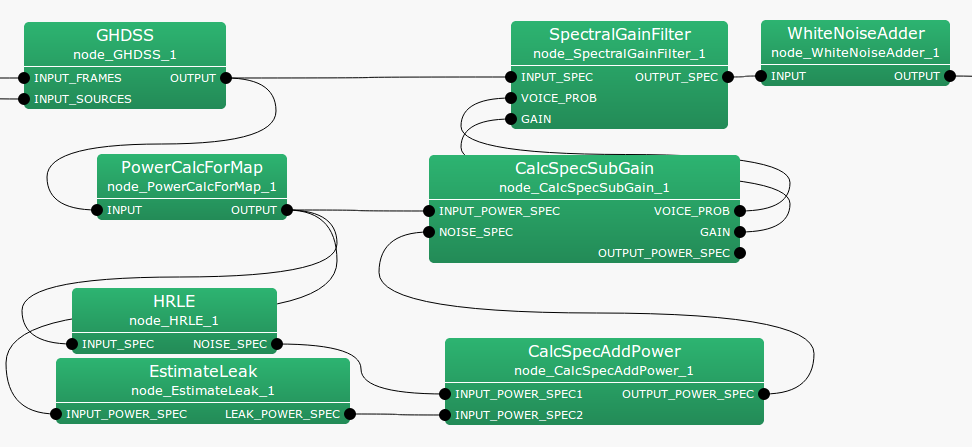## 6.3.10 SpectralGainFilter

### 6.3.10.1 Outline of the node

This node multiplies the separated sound spectrum input by the optimal gain and the probability of speech presence (see PostFilter ) and outputs the result.

### 6.3.10.2 Necessary files

No files are required.

### 6.3.10.3 Usage

When to use

This node is used when obtaining a speech spectrum with suppressed noise from a separated sound spectrum.

Typical connection

Figure 6.67 shows a connection example of SpectralGainFilter . Inputs are 1) separation spectra output from GHDSS 2) the optimal gain, and 3) the probability of speech presence, output from CalcSpecSubGain for example. In the figure, an audio file is created with this node being connected to Synthesize and SaveRawPCM as an example of output.Figure 6.67: Connection example of SpectralGainFilter

### 6.3.10.4 Input-output and property of the node

Input

INPUT_SPEC

: Map<int, ObjectRef> type. The same type as that of the output of GHDSS . A pair containing a sound source ID and the complex spectrum of the separated sound as Vector<complex<float> > type data.

VOICE_PROB

: Map<int, ObjectRef> type. A pair containing a sound source ID and the probability of speech presence as Vector<float> type data.

GAIN

: Map<int, ObjectRef> type. A pair of the sound source ID and the optimal gain as Vector<float> type data.

Output

OUTPUT_SPEC

: Map<int, ObjectRef> type. The same type as the output of GHDSS . A pair containing a sound source ID and the complex spectrum of separated sound as Vector<complex<float> > type data.

Parameter

No parameters

### 6.3.10.5 Details of the node

This node multiplies the input speech spectrum by the optimal gain and the probability of speech presence and outputs a separated sound spectrum with emphasized speech. If the separated sound spectrum input is $X_ n(k_ i)$, the optimal gain is $G_ n(k_ i)$ and the probability of speech presence is $p_ n(k_ i)$, the separated sound for which the input speech is enhanced $Y_ n(k_ i)$ is expressed as follows.

 $\displaystyle Y_ n(k_ i)$ $\displaystyle =$ $\displaystyle X_ n(k_ i) G_ n(k_ i) p_ n(k_ i)$ (108)